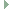OGRE  1.12.1 Object-Oriented Graphics Rendering Engine
Ogre::Matrix4 Class Reference

Transform specialization for projective - encapsulating a 4x4 Matrix. More...

#include <OgreMatrix4.h>Inheritance diagram for Ogre::Matrix4:

## Public Member Functions

Matrix4 ()
Do NOT initialize the matrix for efficiency. More...

Matrix4 (Real m00, Real m01, Real m02, Real m03, Real m10, Real m11, Real m12, Real m13, Real m20, Real m21, Real m22, Real m23, Real m30, Real m31, Real m32, Real m33)

Matrix4 (const Real *arr)

Matrix4 (const Matrix3 &m3x3)
Creates a standard 4x4 transformation matrix with a zero translation part from a rotation/scaling 3x3 matrix. More...

Matrix4 (const Quaternion &rot)
Creates a standard 4x4 transformation matrix with a zero translation part from a rotation/scaling Quaternion. More...

Matrix4 concatenate (const Matrix4 &m2) const

Real determinant () const

void extract3x3Matrix (Matrix3 &m3x3) const

Quaternion extractQuaternion () const

Vector< 3, RealgetTrans () const
Extracts the translation transformation part of the matrix. More...

Matrix4 inverse () const

Matrix3 linear () const
Extracts the rotation / scaling part of the Matrix as a 3x3 matrix. More...

void makeInverseTransform (const Vector3 &position, const Vector3 &scale, const Quaternion &orientation)
Building an inverse Affine3 from orientation / scale / position. More...

void makeTrans (const Vector3 &v)
Builds a translation matrix. More...

void makeTrans (Real tx, Real ty, Real tz)

void makeTransform (const Vector3 &position, const Vector3 &scale, const Quaternion &orientation)
Building a Affine3 from orientation / scale / position. More...

bool operator!= (const Matrix4 &m2) const
Tests 2 matrices for inequality. More...

Matrix4 operator* (Real scalar) const

Matrix4operator= (const Matrix3 &mat3)

bool operator== (const Matrix4 &m2) const
Tests 2 matrices for equality. More...

Realoperator[] (size_t iRow)

const Realoperator[] (size_t iRow) const

void set3x3Matrix (const Matrix3 &mat3)
Assignment from 3x3 matrix. More...

void setScale (const Vector< 3, Real > &v)
Sets the scale part of the matrix. More...

void setTrans (const Vector< 3, Real > &v)
Sets the translation transformation part of the matrix. More...

Matrix4 transpose () const

## Static Public Attributes

static const Matrix4 CLIPSPACE2DTOIMAGESPACE
Useful little matrix which takes 2D clipspace {-1, 1} to {0,1} and inverts the Y. More...

static const Matrix4 IDENTITY

static const Matrix4 ZERO

## Detailed Description

Transform specialization for projective - encapsulating a 4x4 Matrix.

## ◆ Matrix4() [1/5]

 Ogre::Matrix4::Matrix4 ( )
inline

Do NOT initialize the matrix for efficiency.

## ◆ Matrix4() [2/5]

 Ogre::Matrix4::Matrix4 ( Real m00, Real m01, Real m02, Real m03, Real m10, Real m11, Real m12, Real m13, Real m20, Real m21, Real m22, Real m23, Real m30, Real m31, Real m32, Real m33 )
inline

## ◆ Matrix4() [3/5]

 Ogre::Matrix4::Matrix4 ( const Real * arr )
inlineexplicit

## ◆ Matrix4() [4/5]

 Ogre::Matrix4::Matrix4 ( const Matrix3 & m3x3 )
inlineexplicit

Creates a standard 4x4 transformation matrix with a zero translation part from a rotation/scaling 3x3 matrix.

## ◆ Matrix4() [5/5]

 Ogre::Matrix4::Matrix4 ( const Quaternion & rot )
inlineexplicit

Creates a standard 4x4 transformation matrix with a zero translation part from a rotation/scaling Quaternion.

References Ogre::Quaternion::ToRotationMatrix().

## ◆ operator=()

 Matrix4& Ogre::Matrix4::operator= ( const Matrix3 & mat3 )
inline

## ◆ concatenate()

 Matrix4 Ogre::Matrix4::concatenate ( const Matrix4 & m2 ) const
inline

## ◆ operator==()

 bool Ogre::Matrix4::operator== ( const Matrix4 & m2 ) const
inline

Tests 2 matrices for equality.

## ◆ operator!=()

 bool Ogre::Matrix4::operator!= ( const Matrix4 & m2 ) const
inline

Tests 2 matrices for inequality.

## ◆ operator*()

 Matrix4 Ogre::Matrix4::operator* ( Real scalar ) const
inline

## ◆ inverse()

 Matrix4 Ogre::Matrix4::inverse ( ) const

Referenced by Ogre::operator*().

## ◆ makeTrans() [1/2]

 void Ogre::TransformBaseReal::makeTrans ( const Vector3 & v )
inlineinherited

Builds a translation matrix.

## ◆ makeTrans() [2/2]

 void Ogre::TransformBaseReal::makeTrans ( Real tx, Real ty, Real tz )
inlineinherited

## ◆ set3x3Matrix()

 void Ogre::TransformBaseReal::set3x3Matrix ( const Matrix3 & mat3 )
inlineinherited

Assignment from 3x3 matrix.

## ◆ linear()

 Matrix3 Ogre::TransformBaseReal::linear ( ) const
inlineinherited

Extracts the rotation / scaling part of the Matrix as a 3x3 matrix.

## ◆ extract3x3Matrix()

 void Ogre::TransformBaseReal::extract3x3Matrix ( Matrix3 & m3x3 ) const
inlineinherited

## ◆ extractQuaternion()

 Quaternion Ogre::TransformBaseReal::extractQuaternion ( ) const
inlineinherited

## ◆ determinant()

 Real Ogre::TransformBaseReal::determinant ( ) const
inherited

## ◆ makeTransform()

 void Ogre::TransformBaseReal::makeTransform ( const Vector3 & position, const Vector3 & scale, const Quaternion & orientation )
inherited

Building a Affine3 from orientation / scale / position.

Remarks
Transform is performed in the order scale, rotate, translation, i.e. translation is independent of orientation axes, scale does not affect size of translation, rotation and scaling are always centered on the origin.

## ◆ makeInverseTransform()

 void Ogre::TransformBaseReal::makeInverseTransform ( const Vector3 & position, const Vector3 & scale, const Quaternion & orientation )
inherited

Building an inverse Affine3 from orientation / scale / position.

Remarks
As makeTransform except it build the inverse given the same data as makeTransform, so performing -translation, -rotate, 1/scale in that order.

## ◆ operator[]() [1/2]

 Real * Ogre::TransformBase< rows, Real >::operator[] ( size_t iRow )
inlineinherited

## ◆ operator[]() [2/2]

 const Real * Ogre::TransformBase< rows, Real >::operator[] ( size_t iRow ) const
inlineinherited

## ◆ setTrans()

 void Ogre::TransformBase< rows, Real >::setTrans ( const Vector< 3, Real > & v )
inlineinherited

Sets the translation transformation part of the matrix.

## ◆ getTrans()

 Vector<3, Real > Ogre::TransformBase< rows, Real >::getTrans ( ) const
inlineinherited

Extracts the translation transformation part of the matrix.

Referenced by Ogre::Affine3::operator const Matrix4 &().

## ◆ setScale()

 void Ogre::TransformBase< rows, Real >::setScale ( const Vector< 3, Real > & v )
inlineinherited

Sets the scale part of the matrix.

## ◆ ZERO

 const Matrix4 Ogre::Matrix4::ZERO
static

## ◆ IDENTITY

 const Matrix4 Ogre::Matrix4::IDENTITY
static

## ◆ CLIPSPACE2DTOIMAGESPACE

 const Matrix4 Ogre::Matrix4::CLIPSPACE2DTOIMAGESPACE
static

Useful little matrix which takes 2D clipspace {-1, 1} to {0,1} and inverts the Y.

The documentation for this class was generated from the following file: